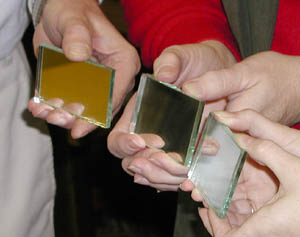# Telescope Technology for Teachers: Human HET - Science and Math TEKS## Challenge 1: Segmented Mirror

Students discover through problem-based learning the cost-effective nature of HET’s mirror array. By solving problems, the students exercise science process skills, apply mathematical knowledge, and test solutions. They discover that instead of a single large mirror, many smaller mirrors acting as one is far less expensive.

 Science TEKS: Challenge 1 6.2 7.2 8.2 IPC Physics Astronomy - Scientific processes. The student use scientific methods during field and laboratory investigations. (A) plan and implement investigative procedures including asking questions, formulating testable hypotheses, and selecting and using equipment and technology; • Given the problem and some information, students must invent their own problem solving proceedure, then formulate and test their hypothesis. (C) Analyze and interpret information to construct reasonable explanations from direct and indirect evidence. • Students create a model array of mirrors out of paper cut outs to help them visualize a solution, and consult a chart of mirror cost vs. diameter (D) Communicate valid conclusions. • Present their model and cost estimate to the class. • The class and presentor ask and answer questions regarding the model. 8.5 - Science concepts.The student knows that relationships exist between science and technology. (A) identify a design problem and propose a solution; (B) design and test a model to solve the problem; (C) evaluate the model and make recommendations for improving the model. 6.5 - Scientific concepts. The student knows that systems may combine with other systems to form a larger system. (A) Identify and describe a system that results from the combination of two or more systems such as in the solar system. Math TEKS: Challenge 1 6.6, 6.7, 7.8 Geometry and Spatial Reasoning 6.8, 7.9, 8.8, 8.10 Measurement 6.11, 7.13 Underlying processes and mathematical tools 7.5, 7.5, 8.5, 8.7 Patterns, relationships, and algebraic thinking

## Challenge 2: Mirror Array

Given string, meter sticks, mirror segments, students form a model of HET’s mirror array.

 Science TEKS: Challenge 2 6.2 7.2 8.2 IPC Physics Astronomy - Scientific processes. The student use scientific methods during field and laboratory investigations. (A) plan and implement investigative procedures including asking questions, formulating testable hypotheses, and selecting and using equipment and technology; (B) Analyze and interpret information to construct reasonable explanations from direct and indirect evidence. (C) Communicate valid conclusions. 8.5 - Science concepts. The student knows that relationships exist between science and technology. (A) identify a design problem and propose a solution; (B) design and test a model to solve the problem; (C) evaluate the model and make recommendations for improving the model. 6.5 - Scientific concepts. The student knows that systems may combine with other systems to form a larger system. (A) Identify and describe a system that results from the combination of two or more systems such as in the solar system. Math TEKS: Challenge 2 6.6, 6.7, 7.8, 8.7 Geometry and Spatial Reasoning 6.8, 7.9, 8.8, 8.9 Measurement 6.11, 7.13, 8.14 Underlying processes and mathematical tools 6.5, 7.4 Patterns, relationships, and algebraic thinking

## Challenge 3: Mirror Alignment

Devise a method to align the mirrors of the model HET mirror array to the center of curvature.

 Science TEKS: Challenge 3 6.2 7.2 8.2 IPC Physics Astronomy - Scientific processes. The student use scientific methods during field and laboratory investigations. (A) plan and implement investigative procedures including asking questions, formulating testable hypotheses, and selecting and using equipment and technology; (B) Analyze and interpret information to construct reasonable explanations from direct and indirect evidence. (C) Communicate valid conclusions. 8.5 - Science concepts.The student knows that relationships exist between science and technology. (A) identify a design problem and propose a solution; (B) design and test a model to solve the problem; (C) evaluate the model and make recommendations for improving the model. 6.5 - Scientific concepts. The student knows that systems may combine with other systems to form a larger system. (A) Identify and describe a system that results from the combination of two or more systems such as in the solar system. Math TEKS: Challenge 3 6.6, 6.7, 7.8 Geometry and Spatial Reasoning 6.11, 7.13, 8.14 Underlying processes and mathematical tools

## Activity 4: Stay Focused

Discover that the focal region of the telescope moves as a star transits HET’s field of view.

 Science TEKS: Challenge 4 6.2 7.2 8.2 IPC Physics Astronomy - Scientific processes. The student use scientific methods during field and laboratory investigations. (A) plan and implement investigative procedures including asking questions, formulating testable hypotheses, and selecting and using equipment and technology; (B) Analyze and interpret information to construct reasonable explanations from direct and indirect evidence. (D) Communicate valid conclusions. 8.5 - Science concepts.The student knows that relationships exist between science and technology. (A) identify a design problem and propose a solution; (B) design and test a model to solve the problem; (C) evaluate the model and make recommendations for improving the model. 6.5 - Scientific concepts. The student knows that systems may combine with other systems to form a larger system. (A) Identify and describe a system that results from the combination of two or more systems such as in the solar system. Math TEKS: Challenge 4 6.7, 7.8 Geometry and Spatial Reasoning 6.8 Measurement 6.11, 6.13, 7.13, 7.15, 8.14 Underlying processes and mathematical tools